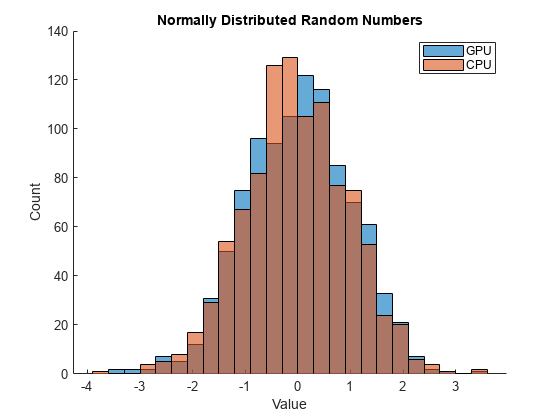# gpurng

Control random number generation for GPU calculations

## Syntax

``gpurng(seed)``
``gpurng('shuffle')``
``gpurng(seed,generator)``
``gpurng('shuffle',generator)``
``gpurng('default')``
``S = gpurng``
``gpurng(S)``
``S = gpurng(___)``

## Description

````gpurng(seed)` sets the starting point, or seed, of the random number generator used in GPU calculations, so that `rand`, `randi`, and `randn` produce predictable sequences of numbers. ```
````gpurng('shuffle')` sets the seed of the random number generator based on the current time so that `rand`, `randi`, and `randn` produce different sequences of numbers after each time you call `gpurng`. ```
````gpurng(seed,generator)` or `gpurng('shuffle',generator)` selects the type of random number generator used by `rand`, `randi`, and `randn`. ```
````gpurng('default')` returns the settings of the random number generator to their default values. The random numbers produced are the same as if you had restarted MATLAB®. The default setting is the `Threefry` generator with seed `0`. ```
````S = gpurng` returns the current state of the random number generator as a structure with fields 'Type', 'Seed', and 'State'. Use this structure to restore the random number generator to the captured settings at a later time with `gpurng(S)`.```
````gpurng(S)` restores the state of the random number generator using settings previously captured with ```S = gpurng```. ```
````S = gpurng(___)` returns the current state of the random number generator as a structure before changing the settings of the seed or generator type.```

## Examples

collapse all

Capture the GPU generator settings, and set the state of the CPU random number generator to match the GPU generator settings. Create predictable arrays of random numbers on the CPU and GPU.

Restore the generator type and seed to their default values on both the CPU and the GPU.

```gpurng('default') rng('default')```

Save the default seed and generator type of the GPU random number generator.

`GPUdef = gpurng`
```GPUdef = struct with fields: Type: 'threefry' Seed: 0 State: [17×1 uint32] ```

Set the CPU random number generator to match the default GPU settings.

`rng(GPUdef) `

Create an array of uniformly distributed random numbers on the GPU.

`rGPU = rand(1,10, 'gpuArray')`
```rGPU = 0.3640 0.5421 0.6543 0.7436 0.0342 0.8311 0.7040 0.2817 0.1163 0.5671 ```

Create an array of random numbers on the CPU.

`rCPU = rand(1,10)`
```rCPU = 1×10 0.3640 0.5421 0.6543 0.7436 0.0342 0.8311 0.7040 0.2817 0.1163 0.5671 ```

The seed and generator type are the same for both the GPU and the CPU, so the arrays are the same.

`isequal(rGPU,rCPU)`
```ans = logical 1 ```

The `gpurng` state does not save the settings for the transformation applied to generate a normally distributed set of random numbers. Even though the seed and the generator type are the same on the GPU and the CPU, the set of normally distributed random numbers is different.

```nGPU = randn(1,1000, 'gpuArray'); nCPU = randn(1,1000); figure hold on histogram(nGPU) histogram(nCPU) legend('GPU','CPU') title('Normally Distributed Random Numbers') xlabel('Value') ylabel('Count') hold off```The statistics of the normal distribution of random numbers are the same on the GPU and the CPU.

By default, the CPU uses the `'Ziggurat'` transformation, while the GPU uses the `'BoxMuller'` algorithm for the `'Threefry' `generator. The only transformation method supported on both the CPU and GPU is the `'Inversion'` transform.

You can change the transformation method on the GPU using `parallel.gpu.RandStream`.

## Input Arguments

collapse all

Random number seed, specified as a nonnegative integer. The seed specifies the starting point for the algorithm to generate random numbers. Specify the seed when you want reproducible results. The default seed is `0`.

Example: `gpurng(7)`

Random number generator, specified as a character vector or string for any valid random number generator that supports multiple streams and substreams. Three random number generator algorithms are supported on the GPU.

KeywordGeneratorMultiple Stream and Substream SupportApproximate Period in Full Precision
`'Threefry'` or `'Threefry4x64_20'`Threefry 4x64 generator with 20 roundsYes2514 (2256 streams of length 2258)
`'Philox'` or `'Philox4x32_10'`Philox 4x32 generator with 10 roundsYes2193 (264 streams of length 2129)
`'CombRecursive'` or `'mrg32k3a'`Combined multiple recursive generatorYes2191 (263 streams of length 2127)

The default generator is `Threefry`.

For more information on the differences between generating random numbers on the GPU and CPU, see Control Random Number Streams on Workers.

Example: `gpurng(0,'Philox')`

Previous random number generator state, specified as a structure previously created using `S = gpurng`.

Example: `S = gpurng` captures the current state of the random number generator, and `gpurng(S)` restores the generator to those settings.

Data Types: `struct`

## Output Arguments

collapse all

Random number generator state, returned as a structure with the fields `'Type'`, `'Seed'`, and `'State'`.

Example: `S = gpurng` captures the current state of the random number generator, and `gpurng(S)` restores the generator to those settings.

Data Types: `struct`

## Version History

Introduced in R2011b

expand all

Behavior changed in R2019a

Behavior changed in R2018a